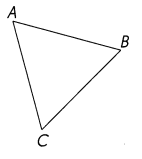# Math in Focus Grade 5 Chapter 13 Answer Key Properties of Triangles and Four-sided Figures

This handy Math in Focus Grade 5 Workbook Answer Key Chapter 13 Properties of Triangles and Four-sided Figures provides detailed solutions for the textbook questions.

## Math in Focus Grade 5 Chapter 13 Answer Key Properties of Triangles and Four-sided Figures

Challenging Practice

This figure is a rhombus and ∠ADO = ∠CDO. Find the measure of ∠DOC.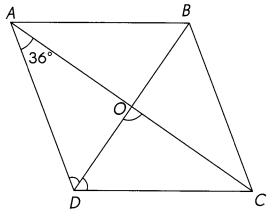∠DOC = 90°
Explanation:
The diagonals of a rhombus bisect each other at right angles (90°)
∠DOC = 90°

Problem Solving

Question 1.
ABCD is a trapezoid in which $$\overline{A D}$$ || $$\overline{B C}$$. Find the measure of ∠CED.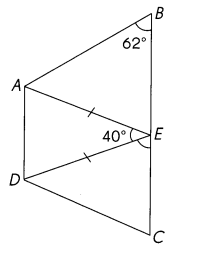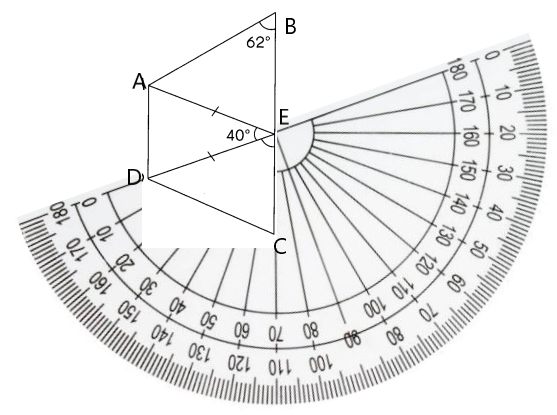Explanation:
With the help of protractor we find the Measure of ∠CED.
∠CED. = 70°

Question 2.
ABCD is a parallelogram and CDEF is a rhombus. Find the measure of ∠ADE.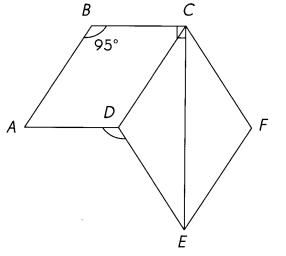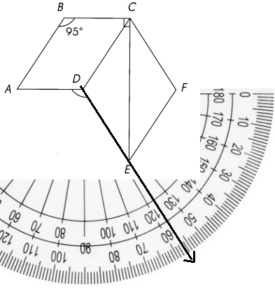Explanation:
By using the protractor we can find the Angle of ADE

Math Journal

Question 1.
A teacher asked her students to sketch and label the angles of a triangle. These are the angle measures that three students chose to draw.
Wayne: 120°, 80°, 10° Ashley: 70°, 28°, 72° Frank: 51 °, 37°, 92°
Will each student be able to draw his or her triangle? Explain your answer.
Wayne: _______
Ashley: ________
Frank: ______
Wayne: 120°, 80°, 10° = 210°
Ashley: 70°, 28°, 72° = 170°
Frank: 51 °, 37°, 92° =  180°
Explanation:
The sum of all the angles of an triangle is equal to 180 degrees
Only Frank can draw the triangle.

Question 2.
What are two ways to identify an isosceles triangle?